# Real Effective Exchange Rate (REER)In this blog, I will discuss the real effective exchange rate (REER). It is the weighted average of a country's currency in relation to a basket of other currencies. This exchange rate is mostly used to determine an individual country's currency value relative to other major currencies. REER is adjusted for the effects of inflation for every currency in the basket, enabling it to be a measure of what can actually be purchased by a currency. REER is used to understand how well a currency is doing with respect to other currencies and also with respect itself in the past. It is an important Quantitative tool that a trader should be cognizant of. Real effective exchange rates are useful in measuring whether a currency has appreciated relative to its trading partners. The real effective exchange rate is volatile over short-term and is not a good indicator for intraday trading, but it can be used in an FX trading strategy with a longer-term perspective. We will demonstrate how to backtest such a strategy in one of our courses on Quantra.

### Calculating The Real Effective Exchange Rate Of A Country

Coming back to REER calculation, a country's REER can be calculated by taking the average of the bilateral real exchange rates (RER) between itself and its trading partners, and then weighing it by using the trade allocation of each partner and then adjusting it for the inflation. This might sound a bit complicated for a beginner, so let us try to understand how to calculate REER with the help of an example.

### Example of Real Effective Exchange Rate

RBI or Reserve Bank of India uses a basket of 36 countries to calculate the real effective exchange rate (REER), but here for the purpose of demonstration, we have used only 6 major trading partners of India. In this example, we will calculate the real effective exchange rate (REER) for the Indian Rupee using the same methodology adopted by the RBI, but with a basket of six currencies: US, Germany, UAE, Saudi Arabia, China, and Hong Kong SAR. The formula for calculating the real effective exchange rate (REER) as given by the RBI: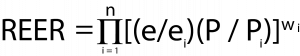Where, n is the number of countries in the basket i is the ‘i’th currency in the basket e is the exchange rate of the Indian rupee against the IMF’s Special Drawing Rights (SDRs) in indexed form ei is the exchange rate of foreign currency ‘i’ against the IMF’s Special Drawing Rights (SDRs) in indexed form wi is the weight attached to the foreign currency ‘i’ Pi is the consumer price index of the country associated with the foreign currency ‘i’ P is India’s consumer price index (CPI), India use the CPI to adjust REER

### Weights

First, let us calculate the weights of each of the 6 currencies. We do this by getting the trade volume data for the six basket countries.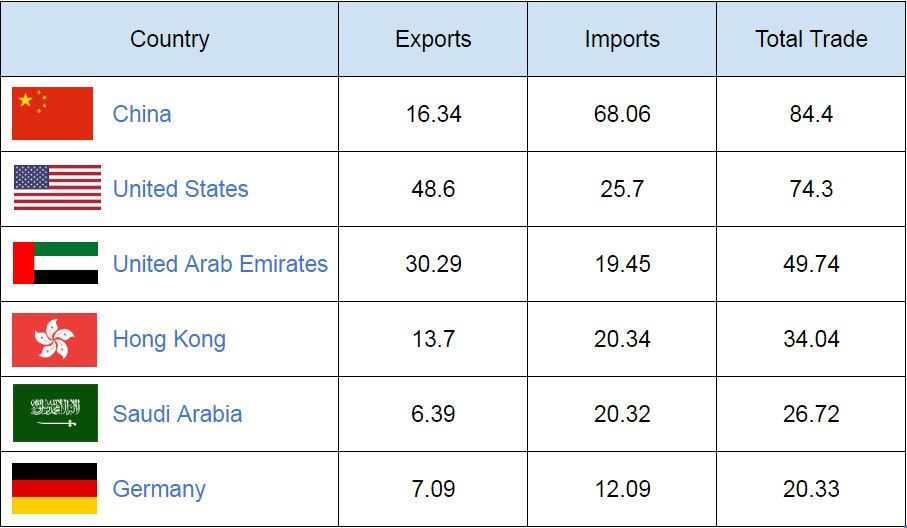* in billions of US dollars for the financial year 2017–18

The total trade with the six countries = (84.4)+(74.3)+(49.74)+(34.04)+(26.72)+(20.33) = \$289.5bn Trade weights for the six countries using the total trade parameter are calculated as follows: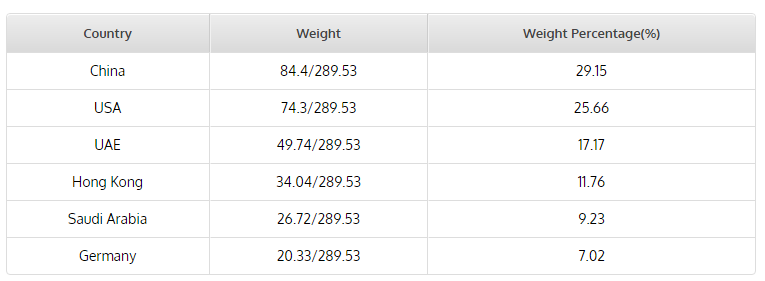### Exchange Rates

Now let us look at the exchange rates of the six basket currencies with respect to the Indian Rupee.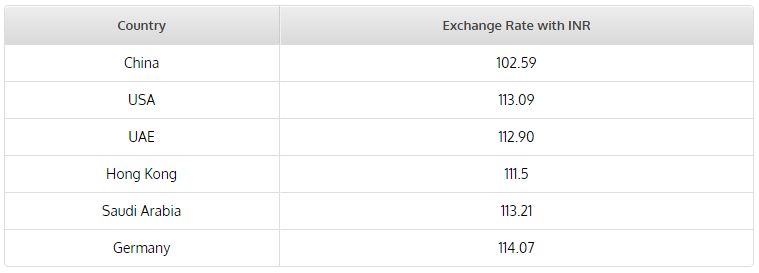* exchange rates as of July 2018 base year Jan 2015

We have considered the EURO/INR as the exchange rate for Germany in this case. While calculating the exchange rate value, the agencies consider an average rate for a predefined period of time. This is done to account for the daily volatile fluctuations in the forex markets. The real effective exchange rate (REER) value is usually released by IMF, central banks and other international agencies with a lag of approximately 3 months.

### Inflation

Let us now look at the inflation data of the 6 basket currencies and India.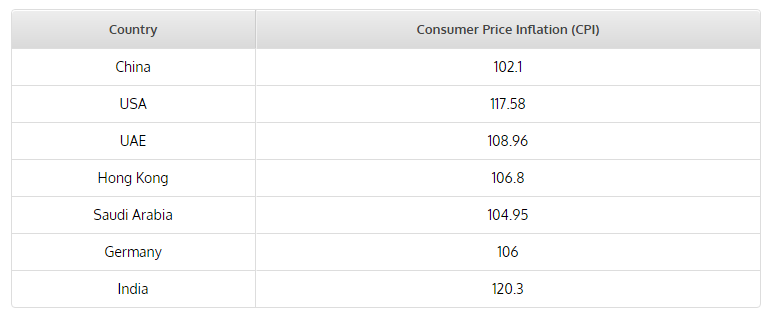* CPI values as of July 2018 scaled base year Jan 2015

### Real Effective Exchange Rate (REER)

Let us calculate the real effective exchange rate (REER) component for China, India’s largest trading partner.

• The exchange rate component= e/e1 = 1/102.59 = 0.00974
• The inflation component = P/P1 = 120.3/102.1 = 1.178
• The product of exchange rate component and inflation component = 0.0094*1.178 =0.0114
• The final REER component = [(e/e1) *(P/P1)]^w1= (0.0114)0.2915= 0.27138

Similarly, once we perform this calculation for the rest of the basket countries, we get the following REER components for each of them as shown below: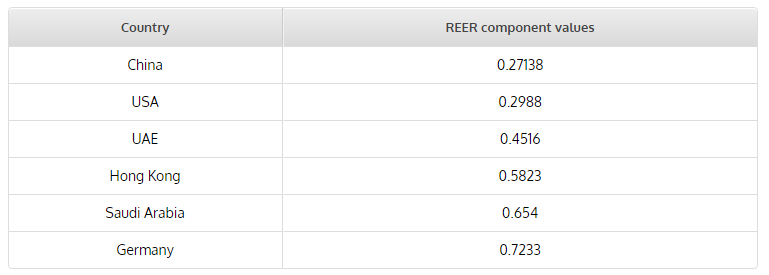Taking the product of all the real effective exchange rate (REER) components we get the total REER for India with respect to the basket of six countries. REER = (0.27138)*(0.2988)*(0.4516)*(0.5823)*(0.654)*(0.7233) = 0.01008 Usually, the REER value is calculated for a particular year is benchmarked to a value of 100 and every subsequent year REER value is computed based on that value. For example, if the REER value that we just calculated is benchmarked to 100. Then the next year, a REER value of 0.01002 is denoted as: REER = 100* 0.01002/0.01008 = 99.404

### Next Step

Hope you have found this blog useful. To learn a real effective exchange rate (REER) based forex trading strategy and to understand the impact of macroeconomic events such as GDP, inflation, interest rates, and balance of trade on the forex markets, you can enroll in the course on value trading in forex market. This course is a blend of various videos, reading materials, IPython notebooks, and Interactive coding exercises to make you understand the concepts in a practical way. The course covers various FX valuation methods such as purchasing power parity (PPP), big mac index (BMI), and real effective exchange rate (REER).

Disclaimer: All investments and trading in the stock market involve risk. Any decisions to place trades in the financial markets, including trading in stock or options or other financial instruments is a personal decision that should only be made after thorough research, including a personal risk and financial assessment and the engagement of professional assistance to the extent you believe necessary. The trading strategies or related information mentioned in this article is for informational purposes only.